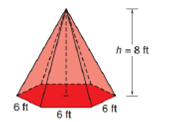Chapter 9.2, Problem 26E### Elementary Geometry for College St...

6th Edition
Daniel C. Alexander + 1 other
ISBN: 9781285195698

#### Solutions

Chapter
Section### Elementary Geometry for College St...

6th Edition
Daniel C. Alexander + 1 other
ISBN: 9781285195698
Textbook Problem
1 views

# The figure below is a regular hexagonal pyramid.a) Find the lateral area L of the pyramid.b) Find the total area T of the pyramid.c) Find the volume V of the pyramid.To determine

(a)

To find:

The lateral area of the pyramid.

Explanation

Given:

A regular hexagonal pyramid as below,

Base side is 6ft and altitude h=8ft.

Properties used:

A pyramid is made by connecting a base to an apex. The base is flat with straight edges, no curves, hence, a polygon and all other faces are triangles.

A regular pyramid is a pyramid whose base is a regular polygon and whose lateral edges are all congruent.

The lateral area L of a regular pyramid with slant height of length l and perimeter P of the base is given by

L=12lP.

In a regular pyramid, the lengths of the apothem a of the base, the altitude h, and the slant height l satisfy the Pythagorean Theorem; that is, l2=a2+h2.

The perimeter of the square is four times the side.

Calculation:

From the given figure

The sides of the square base measure 6ft each and that altitude measures 8ft i.e. h=8ft.

Since, the side of base is 6ft and the angle at the center for each base is 60° which is bisected by the perpendicular so the apothem in the right-angled triangle is given by the perpendicular side i.e. a=side2tan30°

To determine

(b)

To find:

The total area of the pyramid.

To determine

(c)

To find:

The volume of the pyramid.

### Still sussing out bartleby?

Check out a sample textbook solution.

See a sample solution

#### The Solution to Your Study Problems

Bartleby provides explanations to thousands of textbook problems written by our experts, many with advanced degrees!

Get Started

#### Let f be the function defined by f(x)={x2+1ifx0xifx0 Find f(2), f(0), and f(1).

Applied Calculus for the Managerial, Life, and Social Sciences: A Brief Approach

#### In Exercises 7-10, solve for x or y. (3x)2+(74)2=45

Calculus: An Applied Approach (MindTap Course List)

#### The graph of is: a) b) c) d)

Study Guide for Stewart's Multivariable Calculus, 8th